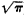# squaring the circle

Also found in: Dictionary, Thesaurus, Financial, Idioms, Wikipedia.
Related to squaring the circle: Doubling the cube

## squaring the circle:

see geometric problems of antiquitygeometric problems of antiquity,
three famous problems involving elementary geometric constructions with straight edge and compass, conjectured by the ancient Greeks to be impossible but not proved to be so until modern times.
.

## Squaring the Circle

the problem of finding a square equal in area to a given circle. To square a circle is to construct a square whose area is equal to that of the circle or to compute the area of the circle with given accuracy.

The attempt was made initially to exactly square the circle with compass and straightedge. The mathematicians of antiquity knew a number of cases when, with the help of these instruments, a curvilinear figure could be transformed into a rectilinear figure of the same area (for example, the lune of Hippocrates). Attempts to square the circle, which continued for thousands of years, were invariably unsuccessful. In 1775 the Paris Academy of Sciences, and later other academies as well, refused to consider work devoted to squaring the circle. Only in the 19th century was a scientific basis given for this refusal: the insolubility of the problem of squaring the circle with compass and straightedge was rigorously established.

If the radius of a circle is equal to r, then the side of the square of the same area is equal to x = r. Thus, the problem reduces to that of graphic multiplication of a given segment (r) by a given number (). However, the graphic multiplication of a segment by a number can be accomplished with compass and straightedge only if the number involved is the root of an algebraic equation with integral coefficients, which is solvable by square roots. Thus, the problem of squaring the circle is equivalent to the problem of determining the arithmetical nature of the number π.

At the end of the 18th century, the irrationality of the number π was established by the German mathematician J. Lambert and the French mathematician A. Legendre. In 1882 the German mathematician F. Lindeman proved that the number π (and that also means) is transcendental, that is, does not satisfy any algebraic equation with integral coefficients. Lindeman’s theorem put an end to attempts to solve the problem of squaring the circle using a compass and straightedge. The problem of squaring the circle becomes solvable if the means of construction are broadened. It was already known to Greek geometers that squaring the circle could be accomplished using transcendental curves; the first solution of the problem of squaring the circle was accomplished by Dinostratus (fourth century B.C.) with the help of a special curve—the quadratrix. On the problem of finding an approximate value for the number π, see the article pi.

### REFERENCES

O kvadrature kruga (Arkhimed, Giuigens, Lambert, Legendre). Sprilo-zheniem istorii voprosa, 3rd ed. Moscow-Leningrad, 1936. (Translated from German.)
Struik, D. J. Kratkii ocherk istorii matematiki, 2nd ed. Moscow, 1969. (Translated from German.)

## squaring the circle

[′skwer·iŋthə ′sər·kəl]
(mathematics)
For a circle with a specified radius, the problem of constructing a square that has the same area as the circle.
Site: Follow: Share:
Open / Close